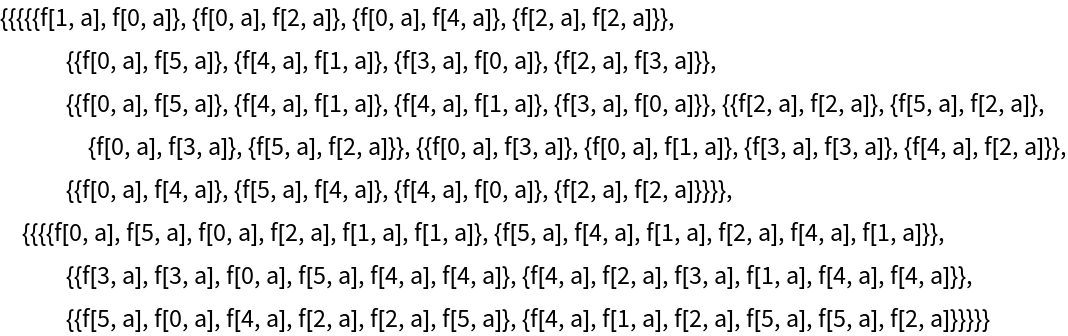#Function Repository Resource:

A version of MapThread that allows for ragged arrays and for lists with unequal depth

Contributed by: Sander Huisman
 ResourceFunction["GeneralizedMapThread"][f,{{a1,a2,…},{b1,b2,…},…}] gives {f[a1,b1,…],f[a2,b2,…],…}. ResourceFunction["GeneralizedMapThread"][f,{expr1,expr2,…},n] applies f to the parts of the expri at level n. ResourceFunction["GeneralizedMapThread"][f] represents an operator form of ResourceFunction["GeneralizedMapThread"] that can be applied to an expression.

## Details and Options

If the lists do not have equal length, the smallest lists are made equal length by making copies using ConstantArray.

## Examples

### Basic Examples

Have the second argument be a constant:

 In:=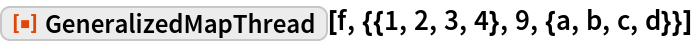Out=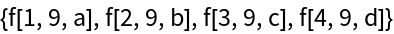It works on expressions with unequal lengths (“ragged”):

 In:=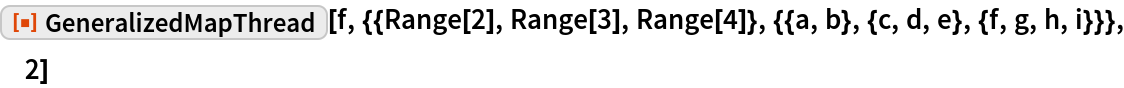Out=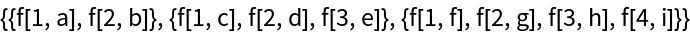Apply f to the corresponding values of associations, copying values where necessary:

 In:=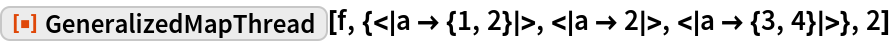Out=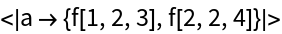### Scope

Apply to a list of lists with varying depths and lengths:

 In:=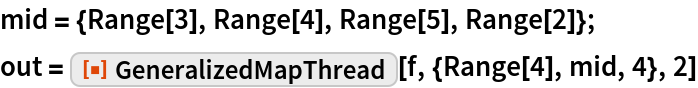Out=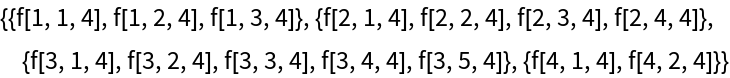The final results are ragged:

 In:=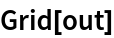Out=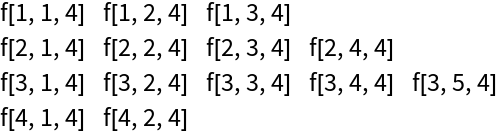Apply at level 1 means that {a,b} from the final list is considered a single value:

 In:=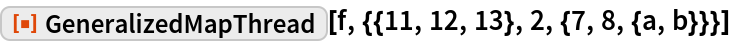Out=Apply at level 2 means interpreting {a,b} as separate values, and copying all the other values:

 In:=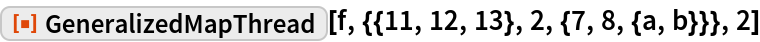Out=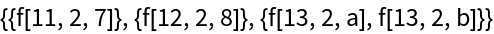In:=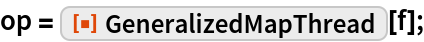Use the operator on some data:

 In:=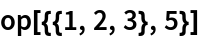Out=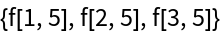### Applications

Subtract the vector {a,b} from a list of vectors:

 In:=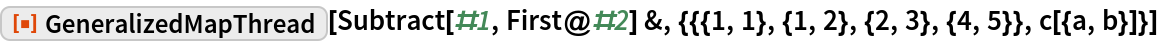Out=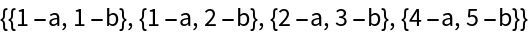Apply a list of functions to a single argument without pure functions:

 In:=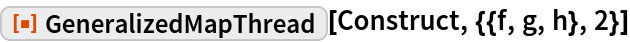Out=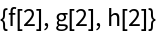This can also be achieved using Through:

 In:=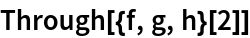Out=### Properties and Relations

When the arrays are of equal length, MapThread and GeneralizedMapThread give the same result:

 In:=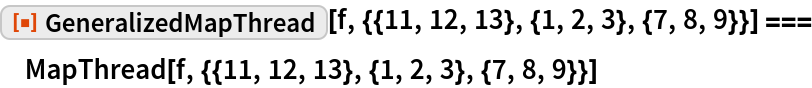Out=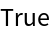MapThread cannot handle lists with elements of unequal length (“ragged”):

 In:=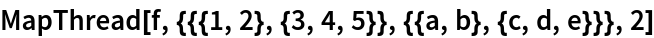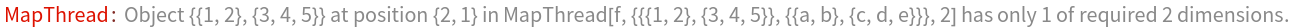Out=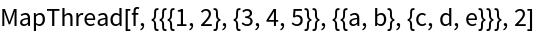In:=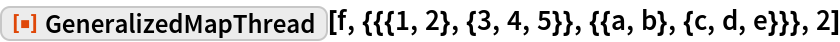Out=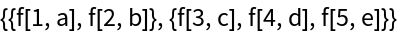Thread can handle arrays with different depths:

 In:=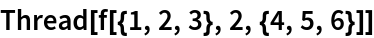Out=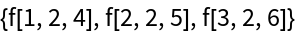In:=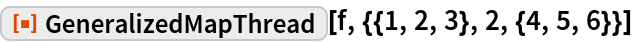Out=### Possible Issues

Associations must have the same keys:

 In:=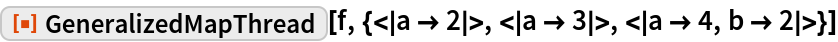Out=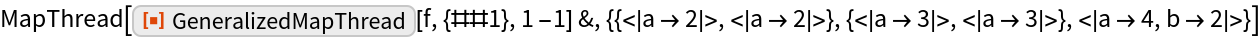Associations and lists cannot be mixed:

 In:=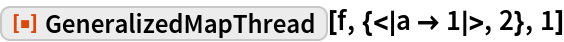Out=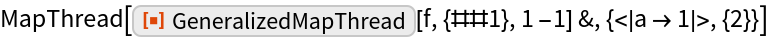Values with another head, such as List or Association, or an incorrect length will get copied:

 In:=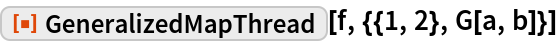Out=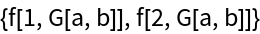For the case of unequal length, the longest list is taken as the actual length. Here the last list is the longest; the first list is copied:

 In:=Out=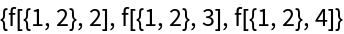Here the first list is the longest; the last list is copied:

 In:=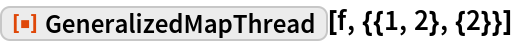Out=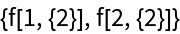An extra bracket around the second list will make it the shortest:

 In:=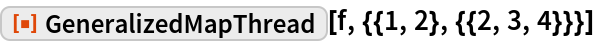Out=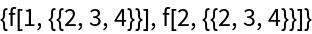One can also give it a different head, which prevents it from being interpreted as a list:

 In:=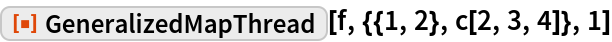Out=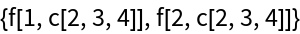### Neat Examples

Interpret the entries as three levels deep, copying them all:

 In:=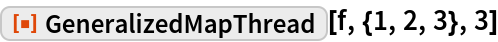Out=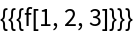Create a complex ragged array with different depths:

 In:=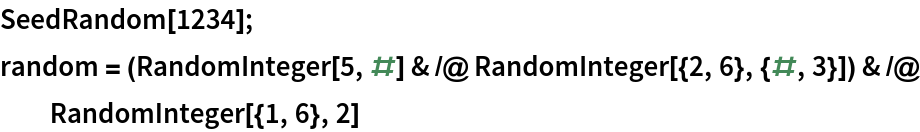Out=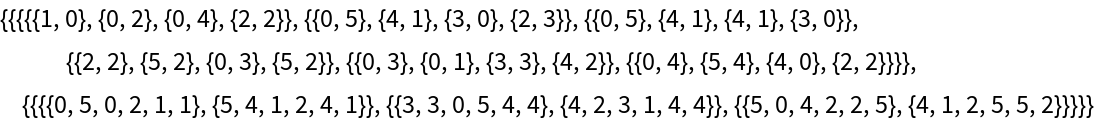Apply the function f five levels deep, with the second argument set constant to a:

 In:=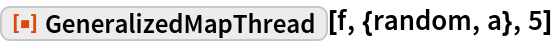Out=